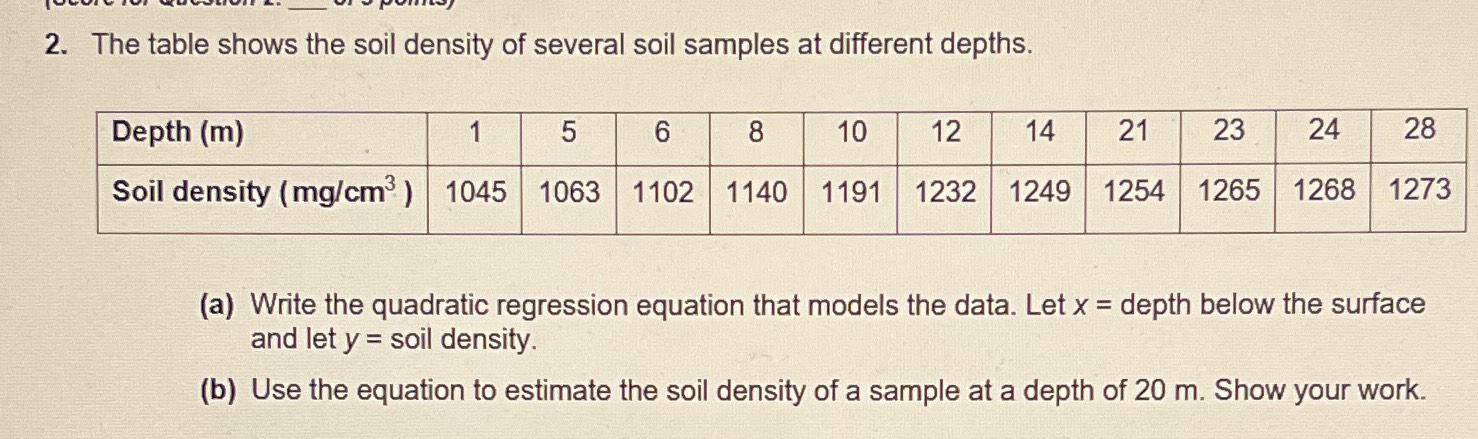### ¿Todavía tienes preguntas de matemáticas?

Pregunte a nuestros tutores expertos
Algebra
Pregunta2. The table shows the soil density of several soil samples at different depths.

(a) Write the quadratic regression equation that models the data. Let $$x =$$ depth below the surface and let $$y =$$ soil density. (b) Use the equation to estimate the soil density of a sample at a depth of $$20 m$$ . Show your work.

$$y= -0.5x^2+ 23.4x+ 996$$